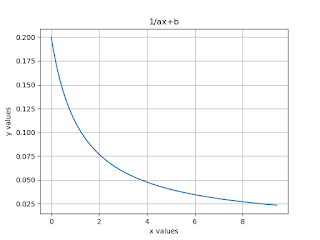## Program to Plotting graph of 1/ax+b?

In this section, we discuss How to Plotting the graph of 1/ax+b using Python Libraries. So in this case, We used Numpy (Numerical Python) and Matplotlib for plotting the graph of the equation. Let's take a look at how to Plotting the graph of 1/ax+b in Python Programming.

## Plotting Graph of 1/ax+b:-

Python Code:
import numpy as np
from matplotlib import pyplot as plt
x = np.arange(
0,3*np.pi,0.01)
a =
4
b = 5
y = 1/(a*x+b)
plt.plot(x,y)
plt.xlabel(
'x values')
plt.ylabel(
'y values')
plt.title(
'1/ax+b')
plt.grid(
True)
plt.show()

Output:Program to Plotting graph of 1/ax+b